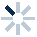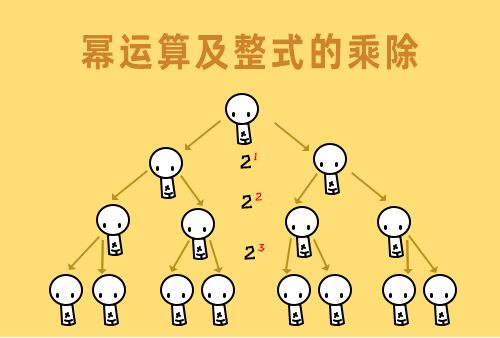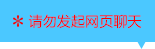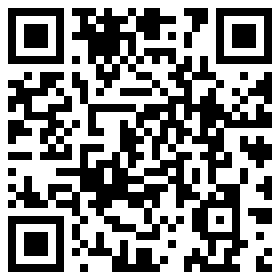| | |

||
APP端下载AndroidiPhone
|
￥0

|
3871人点赞
304920人已学习
|

3天无理由退款

3天无理由退款：退款将以超级币形式退至您的超级课堂学习账户，便于您重新选购其他课程。恶意退款将被冻结账号。

• 1、二次根式的定义，把形如$\sqrt{a}$，$a\geq 0$的式子叫做二次根式，判断二次根式主要抓住两点，二次根号和被开方数非负。
2、 $b\sqrt{a}$这样的形式也是二次根式，它是表示$b$与$\sqrt{a}$的乘积，不是$b$与$\sqrt{a}$的和。
3、 二次根式要有意义，必须使得被开方数（或式子）非负，即$\sqrt{a}$要有意义，必须满足$a\geq 0$。当二次根式与分式同时出现时，还要考虑分母不为$0$。
4、 总结初中阶段的三种非负数形式：平方型，绝对值型，根号型。
• 1、先开方再平方表示为：$\sqrt{a}$的平方等于$a$，$a\geq 0$。
2、 先平方再开方表示为：当$a\geq 0$时，$a$平方的开方等于$a$。；当$a＜0$时，$a$平方的开方等于$-a$。
3、 一个数，先平方再开方，结果是它的绝对值。
• 1、我们认识了二次根式之积的算术平方根具有的拆分及合并性质：$\sqrt{ab}=\sqrt{a}\sqrt{b}$或者$\sqrt{a}\sqrt{b}=\sqrt{ab}$同时切记，都必须满足$a\geq 0$且$b\geq 0$的条件。
• 1、化简二次根式，必须满足两个条件，一被开方数中不含分母，二被开方数中不含能开尽方的因数或因式。比如$\sqrt{200}$化简成$10\sqrt{2}$,$\sqrt{96}$化简成$4\sqrt{6}$。把根号下隐藏的能开方的因数，要全部踢出来，拔掉鱼钩下所有的钉子户。
2、 当根号下是含有负号的字母时，这种钉子户最可怕，要格外小心。比如$\sqrt{-x^{3}}=-x\sqrt{-x}$，根号内外负号的奥秘，你要仔细体会。
• 1、​同类二次根式是指最简二次根式的根号部分相同，就是同类二次根式啦。比如$10\sqrt{2}$，$3\sqrt{2}$和$5\sqrt{2}$。$3\sqrt{2xy}$与$-y\sqrt{2xy}$。
2、 同类二次根式的加减法，类似于合并同类项，实数部分加减，根号部分保持不变。
• 1、商的算术平方根性质，倒过来写也成立，但是都必须满足$a\geq 0$且$b\geq 0$的条件。
2、 寻找有理化因式。初中阶段有理化因式主要有三种：(1)$a\sqrt{x}$与$\sqrt{x}$、(2)$a+b\sqrt{x}$与$a-b\sqrt{x}$、(3)$a\sqrt{x}+b\sqrt{y}$与$a\sqrt{x}-b\sqrt{y}$。
3、 分母有理化，就是分子分母同乘以分母的有理化因式。
• 二次根式综合练习

• 1可以证明
• 2超级学员4264657
• 3超级学员4278162
• 4超级学员4314309
• 5超级学员4314610
• 6超级学员4314925
• 7超级学员4316029
• 8超级学员4316407
• 9超级学员4316924
• 10超级学员4317287215620人在学
￥ 88 ￥ 88265517人在学
￥ 12 ￥ 12214273人在学
￥ 88 ￥ 88214571人在学
￥ 63 ￥ 63

• 0
• 点击分享有好礼
••app端下载关注微信号